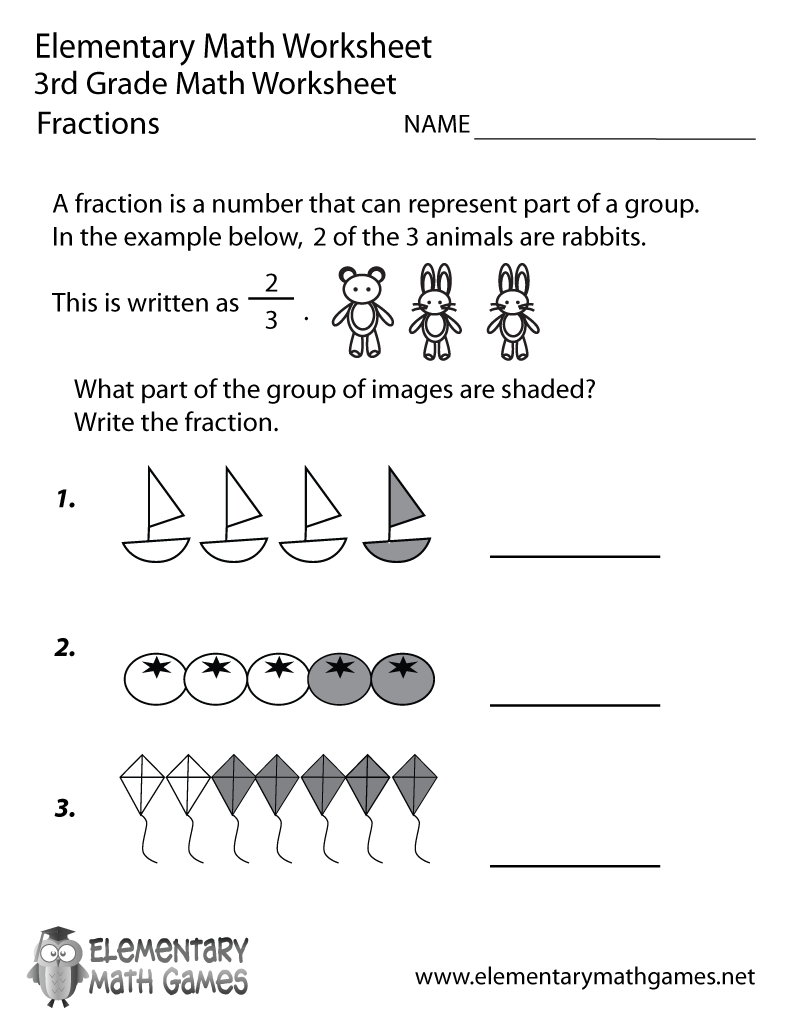# Third Grade Fractions Worksheet## Learn and Practice Fractions

This third grade math worksheet teaches kids about fractions and includes an example. Third graders have to figure out what part of the groups are shaded and then write the correct fraction in the space provided.

Here are the two versions of this free third grade math worksheet: